# 程序员最近都爱上了这个网站  程序员们快来瞅瞅吧！  it98k网:it98k.com

出租广告位,需要合作请联系站长

+关注

## 多元线性回归模型

多元线性回归是一元线性回归的多维运用。在一元线性回归中，我们用自变量系数表示对自变量与因变量之间的解释因素。那么，在多元线性回归中，我们引入多个解释变量，对同一个个体在不同方面的解释进行多维度的衡量。

为了便于理解，我们可以假设有一幢楼进行售卖，用y表示房子的房价，x表示这间房子的不同解释因素，如：面积、位置、采光度、装修等等（影响一间房子的价格绝不仅仅是面积的大小，因此这样的解释是合理的）。

那么，我们就要列举出一个能够综合评判房屋价格的一个数学模型，通过不同维度的计量，从而得到一个能够较全面衡量一间房屋综合价值的数值（类似于国民GDP）。

由此，我们便引入了多元线性回归模型，为了便于观察，我们还引入了基于矩阵运算的模型分析。

通常情况下，一般的多元回归模型写法很多，例如：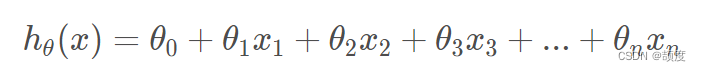这样写太过于繁杂，并且在实际求解的过程中并不能简化我们的运算。为此，引入矩阵运算。

假定x的矩阵为：X=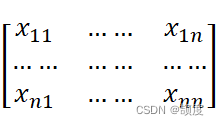，对应的θ的矩阵为：θ=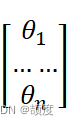在X中添加一个列向量1，得到：X=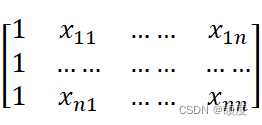通过矩阵运算，可以将hθ（x）=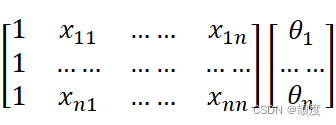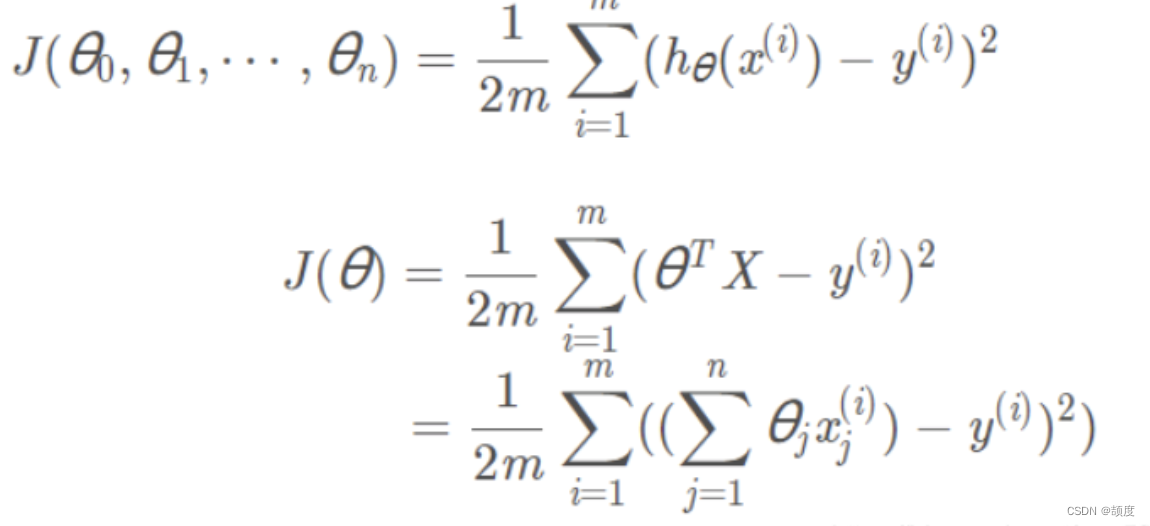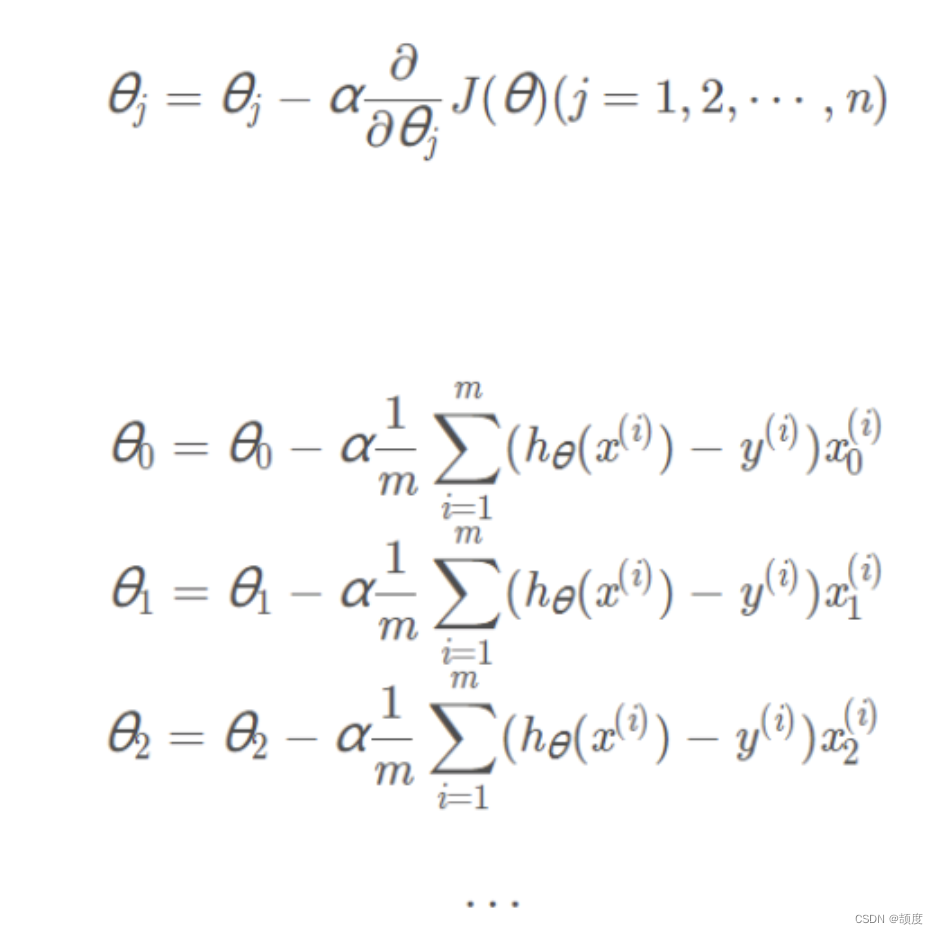Iris 鸢尾花数据集内包含 3 类，分别为山鸢尾（Iris-setosa）、变色鸢尾（Iris-versicolor）和维吉尼亚鸢尾（Iris-virginica），共 150 条记录，每类各 50 个数据，每条记录都有 4 项特征：花萼长度、花萼宽度、花瓣长度、花瓣宽度，可以通过这 4 个特征预测鸢尾花卉属于哪一品种。 这是本文章所使用的鸢尾花数据集： sl：花萼长度 sw：花萼宽度 pl：花瓣长度 pw：花瓣宽度； type：类别：（Iris-setosaIris-versicolorIris-virginica 三类）

部分展示：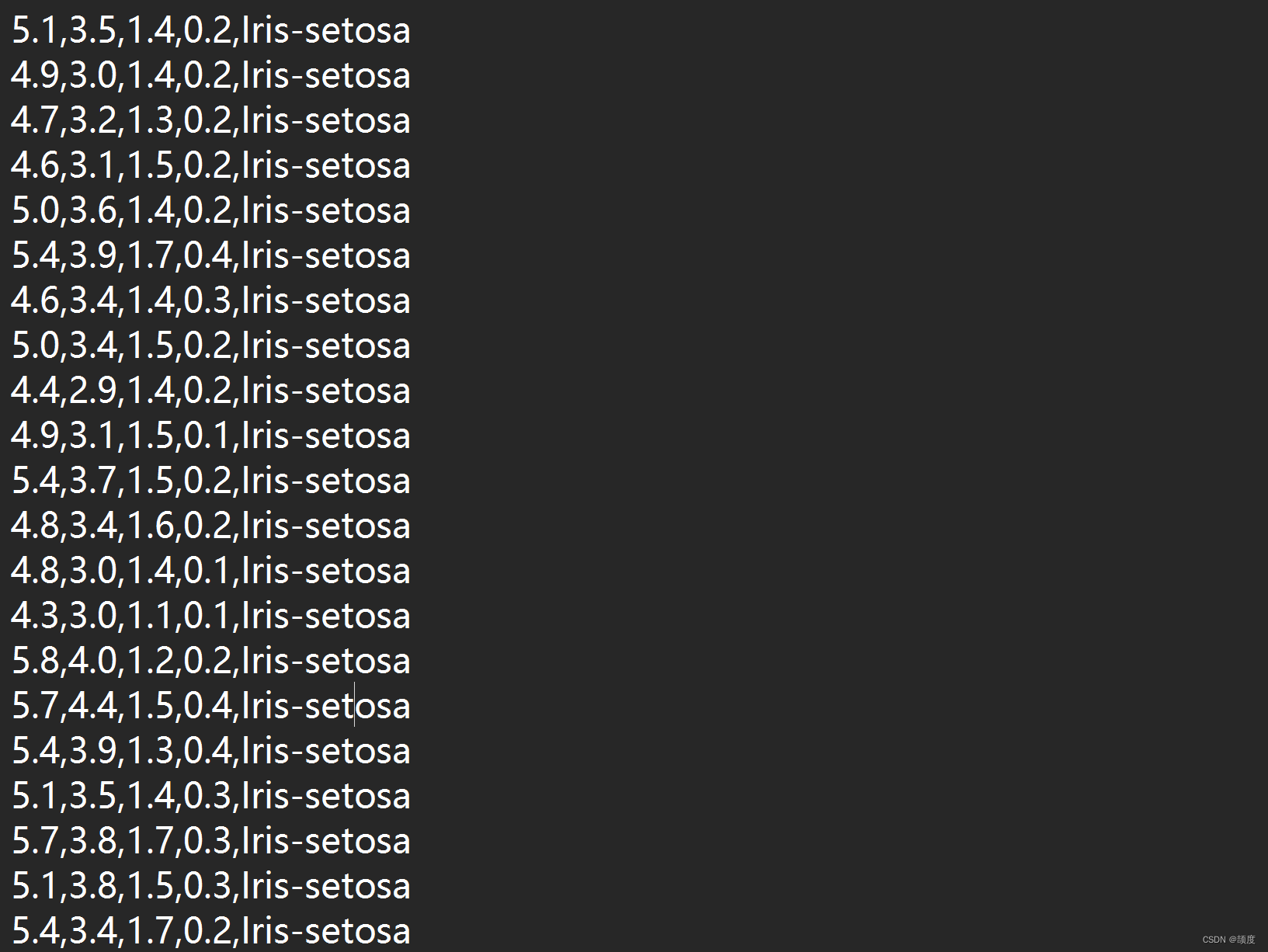``import pandas as pdimport numpy as npimport timeimport randomdef MGD_train(X, y, alpha=0.0001, maxIter=1000, theta_old=None):    '''    MGD训练线性回归    传入:        X       :  已知数据          y       :  标签        alpha   :  学习率        maxIter :  总迭代次数     返回:        theta : 权重参数    '''    # 初始化权重参数    theta = np.ones(shape=(X.shape,))    if not theta_old is None:        # 假装是断点续训练        theta = theta_old.copy()     # axis=1 表示横轴，方向从左到右；axis=0 表示纵轴，方向从上到下    for i in range(maxIter):        # 预测        y_pred = np.sum(X * theta, axis=1)        # 全部数据得到的梯度        gradient = np.average((y - y_pred).reshape(-1, 1) * X, axis=0)        # 更新学习率        theta += alpha * gradient    return theta  def SGD_train(X, y, alpha=0.0001, maxIter=1000, theta_old=None):    '''    SGD训练线性回归    传入:        X       :  已知数据          y       :  标签        alpha   :  学习率        maxIter :  总迭代次数    返回:        theta : 权重参数    '''    # 初始化权重参数    theta = np.ones(shape=(X.shape,))    if not theta_old is None:        # 假装是断点续训练        theta = theta_old.copy()    # 数据数量    data_length = X.shape    for i in range(maxIter):        # 随机选择一个数据        index = np.random.randint(0, data_length)        # 预测        y_pred = np.sum(X[index, :] * theta)        # 一条数据得到的梯度        gradient = (y[index] - y_pred) * X[index, :]        # 更新学习率        theta += alpha * gradient    return theta  def MBGD_train(X, y, alpha=0.0001, maxIter=1000, batch_size=10, theta_old=None):    '''    MBGD训练线性回归    传入:        X          :  已知数据          y          :  标签        alpha      :  学习率        maxIter    :  总迭代次数        batch_size :  没一轮喂入的数据数``
``     返回:        theta : 权重参数    '''    # 初始化权重参数    theta = np.ones(shape=(X.shape,))    if not theta_old is None:        # 假装是断点续训练        theta = theta_old.copy()    # 所有数据的集合    all_data = np.concatenate([X, y.reshape(-1, 1)], axis=1)    for i in range(maxIter):        # 从全部数据里选 batch_size 个 item        X_batch_size = np.array(random.choices(all_data, k=batch_size))        # 重新给 X, y 赋值        X_new = X_batch_size[:, :-1]        y_new = X_batch_size[:, -1]        # 将数据喂入，更新 theta        theta = MGD_train(X_new, y_new, alpha=0.0001, maxIter=1, theta_old=theta)    return thetadef GD_predict(X, theta):    '''    用于预测的函数    传入:        X     : 数据        theta : 权重    返回:        y_pred: 预测向量    '''    y_pred = np.sum(theta * X, axis=1)    # 实数域空间 -> 离散三值空间，则需要四舍五入    y_pred = (y_pred + 0.5).astype(int)    return y_preddef calc_accuracy(y, y_pred):    '''    计算准确率    传入:        y        : 标签        y_pred   : 预测值    返回:        accuracy : 准确率    '''    return np.average(y == y_pred) * 100# 读取数据iris_raw_data = pd.read_csv('iris.data', names  =['sepal length', 'sepal width', 'petal length', 'petal width', 'class'])# 将三种类型映射成整数Iris_dir = {'Iris-setosa': 1, 'Iris-versicolor': 2, 'Iris-virginica': 3}iris_raw_data['class'] = iris_raw_data['class'].apply(lambda x:Iris_dir[x])# 训练数据 Xiris_data = iris_raw_data.values[:, :-1]# 标签 yy = iris_raw_data.values[:, -1]# 用 MGD 训练的参数start = time.time()theta_MGD = MGD_train(iris_data, y)run_time = time.time() - starty_pred_MGD = GD_predict(iris_data, theta_MGD)print("MGD训练1000轮得到的准确率{:.2f}% 运行时间是{:.2f}s".format(calc_accuracy(y, y_pred_MGD), run_time))# 用 SGD 训练的参数start = time.time()theta_SGD = SGD_train(iris_data, y)run_time = time.time() - starty_pred_SGD = GD_predict(iris_data, theta_SGD)print("SGD训练1000轮得到的准确率{:.2f}% 运行时间是{:.2f}s".format(calc_accuracy(y, y_pred_SGD), run_time))# 用 MBGD 训练的参数start = time.time()theta_MBGD = MBGD_train(iris_data, y)run_time = time.time() - starty_pred_MBGD = GD_predict(iris_data, theta_MBGD)print("MBGD训练1000轮得到的准确率{:.2f}% 运行时间是{:.2f}s".format(calc_accuracy(y, y_pred_MBGD), run_time))``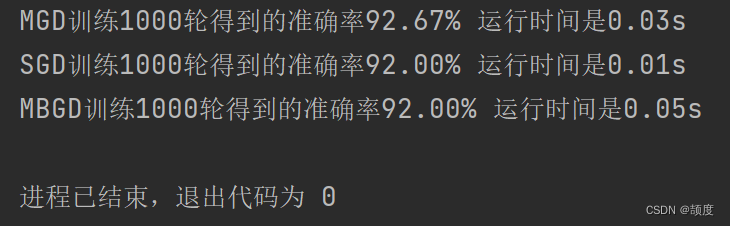16 0

pdf(new) 更多>